﻿ Extreme Value Modelling for Measuring Financial Risk with Application to Selected Philippine Stocks

# Journal of Applied & Computational MathematicsOpen Access

All submissions of the EM system will be redirected to Online Manuscript Submission System. Authors are requested to submit articles directly to Online Manuscript Submission System of respective journal.

# Extreme Value Modelling for Measuring Financial Risk with Application to Selected Philippine Stocks

Velasco AAF* and Lapuz DKP
Department of Mathematics, De La Salle University, 2401 Taft Ave, Malate, Manila, 1004 Metro Manila, Philippines
*Corresponding Author: Velasco AAF, Department of Mathematics, De La Salle University, 2401 Taft Ave, Malate, Manila, 1004 Metro Manila, Philippines, Tel: 639266385740, Email: [email protected]

Received Date: Jul 10, 2018 / Accepted Date: Jul 16, 2018 / Published Date: Jul 23, 2018

Keywords: Extreme value theory; Generalized Pareto distribution; Peaks over threshold; Value at risk; Conditional value at risk; Return level; Backtesting

#### Introduction

Extreme value theorem (EVT) plays a significant role in the financial industry when it comes to estimating extreme events, which makes it useful in measuring risks [1,2]. Extreme events in finance are those events that occur with low probabilities of at most 0.05. Several studies on predictive performance of various values at risk (VaR) methods found EVT-based methods to be particularly accurate .

Fat tail distribution is a statistical distribution phenomenon. Its circumstance is commonly observed in financial returns data . Manifestations of this are events which are less likely to occur but with high probability of devastating consequences when they take place . Traditional method for financial risk measure often has an assumption of normality in the distribution. However, in most cases the data has fat tails and does not make true of the required assumption . Therefore, in measuring financial risk, analysts need to apply a method without presumption of normal distribution . EVT method may be used as it does not put emphasis on the distribution of the data. The drew attention to the insufficiency of the normal distribution for modeling the marginal distribution of returns as their heavy-tailed [8,9] disposition has a wider range than normal distribution. Few gather around the center and more populate the extremes either far above or far below the average [10,11]. Therefore, commonly, there is a sharp peakedness and heavy-tailedness in the distribution of price changes or returns [12,13].

In correspondence several market risk models have been developed, researchers have noted some common issues in risk measurement [14,15]. These issues typically arise from the disagreement between model assumptions and actual observations and/or empirical studies on financial data. Among these issues are eqn. (1) the non-normality or fat-tailedness of price distribution assets, eqn. (2) the presence of serial correlation and heteroscedasticity in financial time series [16,17], and eqn. (3) the problem of whether to model the entire distribution or only the tails. To address these issues, Suaiso and Mapa proposed the use of EVT approach in measuring market risk.

EVT is a technique for risk measurement especially when the concern is the tail of a probability distribution . It gives the best available models to predict extreme events as it usually does not underestimate tail risk because it does not assume normality which traditional methods require [19,20]. In addition, EVT has methods in estimating extreme conditions because statistical methods illustrating common central values of observed variables could fail in describing such extreme behaviors .

What makes the EVT appealing is the fact that the nature of the asymptotic distribution of returns does not necessarily depend on the exact distribution of returns [22-24]. Additionally, EVT-based estimates of VaR directly concentrates on the tails of the distribution, thus avoiding a major flaw of other parametric approaches whose estimates are somehow biased by the emphasis they give to the central part of the distribution which leads to underestimating extremes and outliers. These extremes and outliers are of interest when calculating VaR . Also, EVT allows one to concentrate on each of the two tails of the distribution independently , thus, allowing a flexible approach which can take skewness (a typical feature in financial time series) of the underlying distribution into account. The reveal that the different daily return distributions have different moment properties at their left and right tails. As some studies concluded, the left and right tails behave differently, and thus should be treated separately when estimating risk measures .

There are two broad methods of applying EVT [27,28]. The first of which is based on the generalized extreme value distributions known as the block maximum model (BMM) approach. The second one is based on the generalized Pareto distribution (GPD) and is known as the peaks over threshold (POT) approach . The block maxima method is the conventional EVT approach. It subdivides the sample into several blocks, from which a maximum can be drawn from each block. The distribution of the block maxima is determined by fitting the generalized extreme value (GEV) to the set of block maxima. One caveat in this approach is the choice of block size . The more flexible approach to estimation of risk measures such as the [31-33] VaR is by using price changes greater than a chosen high threshold (exceedances) which known as the POT approach. Nortey et al.  stated that the POT approach developed by Pickands uses data more efficiently compared to the BMM approach.

The POT approach of EVT offers two methods . These are the unconditional or static EVT model, which is the straightforward application of POT to the rates. The other is the conditional or dynamic EVT model, which applies POT to the residuals of the fitted ARMAGARCH model to account for the presence of serial correlation and heteroscedasticity .

In a study by Suaiso and Mapa , the conditional and unconditional POT approach of the EVT in measuring market risk is compared with traditional VaR methods such as RiskMetrics and ARGARCH type models. The relative size, accuracy, an efficiency of the models are assessed using mean relative bias, backtesting, likelihood ratio tests, loss function, mean relative scaled bias, and computation of market risk charge. Their findings show that the conditional EVT model can capture market risk conservatively, accurately, and efficiently. Comparing the two EVT models, the conditional model is better than the unconditional model as the former can address some issues in risk measurement and effectively capture market risks .

Nortey et al.  studied the application of EVT in modeling the Ghana stock exchange index. The conditional EVT was applied due to the observed volatility in the returns series. Results showed that the POT approach of EVT, which fits a GPD model to the excesses above a threshold, can be very efficient in the modeling of extreme events in the stock market. A similar study was performed by Magnou  by applying EVT techniques to the Uruguayan Pension Fund returns which supports the findings of Nortey et al.  on the efficiency of EVT in financial risk application .

This study aims to measure financial risk associated with selected Philippine stocks under the framework of EVT. The objectives of the study are (1) to introduce and illustrate the Peaks Over Threshold (POT) approach of EVT in modeling tail returns of selected Philippine stocks, (2) demonstrate the use of semi-automatic threshold selection in R, (3) calculate risk measures such as value at risk (VaR), conditional value at risk (CVaR), and return level, (4) compare the risk measures under EVT with those computed under the normal distribution assumption and against the empirical result, (5) compute the threshold of the Basel Committee three-zone approach for backtesting at the 99% and 95% coverage levels for sample size 265; and (6) assess the accuracy of VaR models under EVT using the computed backtesting thresholds and compare with the accuracy of VaR models under normal distribution assumption.

It should be noted that this paper does not intend to discuss the causes of extreme events.

Instead, it will take a statistical perspective on the marginal tail distribution of the selected Philippine stocks’ fluctuations.

#### Conceptual Framework

The methods used in measuring financial risk for the stocks of Banco de Oro (BDO), Megaworld Corporation (MEG), SM Investments Corporation (SM), Semirara Mining and Power Corporation (SCC), and Universal Robina Corporation (URC) using the daily closing prices which were obtained from the Philippine Stock Exchange (PSE) are presented and discussed.

Autoregressive moving average (ARMA)

The process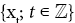is an autoregressive moving average process of order (p,q), denoted by xt~ARMA(p,q) if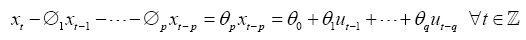where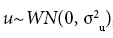, and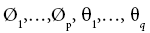are p+q constant and the polynomials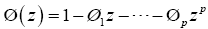and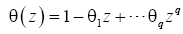have no common factors .

Augmented Dickey-Fuller unit root test

Before ARMA modeling, a series must be fairly stationary. To test for stationary, augmented Dickey-Fuller (ADF) test may be employed. When the time series is flat and potentially slow-turning around zero, the test equation of ADF is given by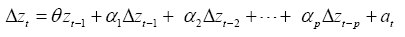where the number of augmenting lags (p) is determined by minimizing the Schwartz Bayesian information criterion or minimizing the Akaike information criterion or lags are dropped until the last lag is statistically significant .

Box-Ljung test

Box-Ljung test is a diagnostic tool used to test the lack of fit of a time series model. The test is applied to the residuals of a time series after fitting an ARMA (p,q) model to the data. The test examines m autocorrelations of the residuals. If the autocorrelations are very small, we conclude that the model does not exhibit significant lack of fit. The Box-Ljung Q-test is a more quantitative way to test for autocorrelation at multiple lags jointly (Ljung and Box, 1967). The null hypothesis for this test is that the first m autocorrelations are jointly zero.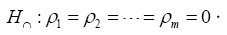The choice of m affects test performance. If N is the length of the observed time series, choosing m=In(N) is recommended for power . Multiple values of m may also be tested. If seasonal autocorrelation is possible, consider testing at larger values of m such as 10 or 15. The Box-Ljung test statistic is given by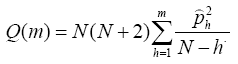General autoregressive conditional heteroscedasticity (GARCH)

The academic literature on modelling value at risk (VaR) and conditional value at risk (CVaR) indicates that a successful approach for modelling the high quintiles of the portfolio loss distribution is to combine a GARCH model with the EVT . The GARCH part is responsible for capturing the dynamics of volatility while EVT provides a model for the behaviour of the extreme tail of the distribution.

The GARCH (p,q) process is defined as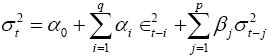where the variance at time t is denoted bywhile εt is a time series that is equal to σt ωt where ωt is discrete white noise with zero mean and unit variance and αi and βj are parameters of the model for which α0>0,αi ≥ 0,βj ≥ 0, i=1,…, q j=1,…,p.

Lagrange multiplier test

The Lagrange Multiplier (LM) test is a general principle for testing hypotheses about parameters in a likelihood framework. The hypothesis under test is expressed as one or more constraints on the values of the parameters. To perform an LM test only estimation of the parameters subject to the restrictions is required. The LM testing principle has found wide applicability to many problems of interest in econometrics . Lagrangian Multiplier (LM) test is performed to examine the data for the presence of autoregressive conditional heteroscedastic (ARCH) effects and to indicate the presence of significant ARCH effects in the data .

Extreme value theory

The discussion of the POT approach is lifted from Nortey et al. . Given a set of observations X1, X2,…,Xn with cumulative distribution function F(x) and a pre-determined threshold u, we are interested in the conditional distribution of the exceedances with respect to the threshold.

The POT method considers the distribution of exceedances over this threshold. As illustrated in Figure 1, an unknown distribution function F of a random variable X is considered. The distribution function Fu for values of x above a certain threshold u is estimated Magnou . The exceedances are modeled using GPD with distribution function denoted by Fu(y), where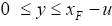.

If Xi>u, for any i=1,2,…, n, an exceedance is said to occur. Hence, we can define Y=Xi-u as the exceedances with respect to u. The corresponding distribution function, known as the conditional distribution function of excesses, Fu(y) defined as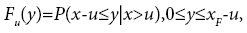Where xF<∞ is the right end-point of F. We have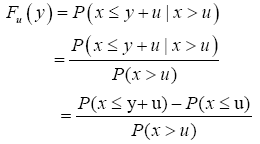Hence, since x=u+y for X>u, F expressed in terms of Fu gives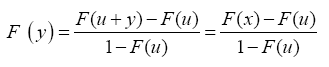Clearly, as the bulk of this observations lie in the range [0,u], the estimation of the distribution within this range is quite straightforward. However, a challenge in the estimation of the distribution above is in the presence of only a few numbers of observations in this range. The estimation of this conditional distribution function of excesses was proposed in Theorem 1.

Theorem 1

Balkema and Haan , For a large class of underlying distribution F, the excess distribution function Fu can be approximated by GPD for increasing threshold u.

As the threshold u becomes large and if the underlying distribution of the returns series belongs to the maximum domain of attraction of the Generalized Extreme Value (GEV) distribution, the distribution function of the exceedances over the threshold has approximately a GPD.

Hence, for a large class of underlying distributions F and a high threshold u, the conditional distribution of excesses Fu(y) is well approximated by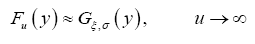Where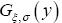is the GPD which is given by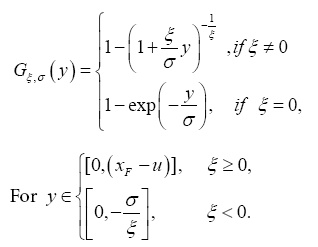In the given GPD model, ξ is the shape parameter or the tail index while σ is the scale parameter. The value of the scale parameter σ gives an idea of the behavior of the tail of the distribution with a large value indicating a very heavy tail. Indicated that generally, an upper tail for financial losses cannot be fixed. Because of this, only distributions with shape parameter ξ≥0 are suited to model financial return series.

The GPD model can be expressed as a function of x by defining x=u+y which gives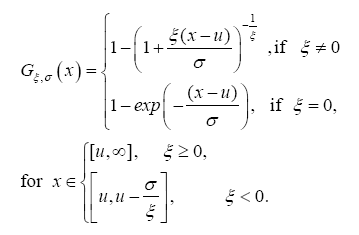Setting u=0 and σ=1, the resulting equation is also known as the standard GPD

The mean excess function for the GPD with parameter ξ<1 is also defined as

e(u)=E(X-u|X>u), where X-u|X>u~GPD(ξ,σ)

and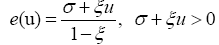This function gives the mean of the excesses of X over varying values of the threshold u.

The shape parameter ξ provides information on the tail distribution. If ξ>0 the tail of the GPD is Pareto and if ξ=0 the tail is exponential. In addition, if ξ<0 the GPD has a finite right endpoint. The two approaches mostly employed in the parameter estimation of the GPD are the maximum likelihood estimation (MLE) method and the method of Probability Weighted Movements (PWM). To estimate the parameters of the GPD that will be fitted to the exceedances, MLE will be considered because it produces smaller standard deviation .

Risk measures

The issues of concern to most risk managers and financial analysts are the events that occur under extreme market conditions. These are events which have a tendency to produce huge unexpected losses that can lead to bankruptcy.

Since its introduction in the 1990s, value at risk (VaR) has become a standard risk measure in the practice of finance. It provides a threshold of the portfolio loss distribution such that losses higher than the threshold occur with a given probability; typical choices include 1% or 5%. There are two ways in which EVT can be applied to VaR modeling. It is either done by directly applying EVT on the return series or by first running a GARCH model to account for clustering of volatility effect. The conditional approach through GARCH relies on cleaning the clustering of the extremes first through a GARCH model and EVT is applied to the residuals time series .

As a result of the observed volatility in the daily returns data, the conditional EVT approach was preferred for the study. A similar realization was made by Polakow and Seymour  when they compared the conditional and unconditional approaches in the modeling of volatile South African stock market, and the conditional approach provided better results compared to the unconditional approach.

The discussion on the derivation of risk measures is from Nortey et al.  and Magnou . Assuming a GPD function for the tail distribution,VaRα,CVaRα, and γM can be defined as a function of the GPD parameters.

The VaR of a financial portfolio at a confidence level, 0<α<1 can be defined as the smallest number l such that the probability of a loss L exceeding l over a certain time horizon is smaller than or equal to α . Thus, it is given as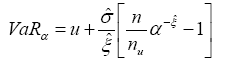(1)

Another measure of risk, conditional value at risk (CVaR), which is the average losses beyond VaR, has gained popularity. According to the Basel committee on banking supervision, it is more informative than VaR and has better properties for an extended analysis of the application of VaR for risk measurement in the context of regulation.

The CVaR is defined as the expected size of a loss given that the loss exceeds VaRα and is given by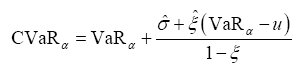(2)

CVaR, as opposed to VaR, is a coherent risk measure in the sense that it satisfies the properties of monotonicity, sub-additively, homogeneity, and translational invariance McNeil et al. .

The return level,γm, that is exceeded on average once every m observations is given by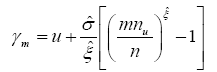(3)

For presentation, it is often more convenient to give return levels on an annual scale so that the M-year return level is the level expected to be exceeded once every M years. If there are k observations per year, this corresponds to the m-observation return level, where m=M×k.

Hence, the M-year return level is defined by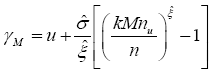(4)

Backtesting

The Basel Committee on Banking Supervision provides a forum for regular cooperation on banking supervisory matters. Its objective is to enhance understanding of key supervisory issues and improve the quality of banking supervision worldwide.

The Basel Committee on Banking Supervision (1996) developed a backtesting framework based on the method adopted by many of the banks that use internal market risk measurement models. The objective is to test the quality and accuracy of the VaR model. These backtesting programs typically consist of a periodic comparison of daily VaR measures with the subsequent daily profit or loss. The VaR measures are intended to be larger than all but a certain fraction of the trading outcomes, where that fraction is determined by the significance level of the VaR measure. Comparing the risk measures with the trading outcomes means counting the number of times that the risk measures were larger than the trading outcome. The fraction covered can then be compared with the intended coverage level to gauge the performance of the risk model. In interpreting the results of backtesting, the Basel Committee introduced the three-zone approach found in Table 1.

Zone Number of exceptions Cumulative probability (%)
Green 0 8.11
1 28.58
2 54.32
3 75.81
4 89.22
Yellow 5 99.88
6 98.63
7 99.60
8 99.89
9 99.97
Red ≥10 99.99

Table 1: Basel Committee three-zone approach for backtesting.

The table defines the green, yellow, and red zones that will be used to assess backtesting results. The results shown in the table are based on a sample of 250 observations. For other sample sizes or coverage level, the yellow zone begins at the point where the cumulative probability equals or exceeds 95%, and the red zone begins at the point where the cumulative probability equals or exceeds 99.99%. The cumulative probability is simply the probability of obtaining a given number or fewer exceptions in a sample of 250 observations when the true coverage level is 99%.

If the model falls into the green zone, then there is no problem with the quality or accuracy of the model. The yellow zone is an ambiguous zone and the conclusion of model inaccuracy is not definite. If the model falls into the red zone, there is a high probability that the model is inaccurate .

#### Methodology

This presents the various methods and techniques employed in this study. The data used in the study as well as various assessment methods to describe the data. Illustrates producing a standardized independent and identically distributed (iid) series by fitting AR-GARCH models. It presents the application of EVT in modeling the tails of the iid series. Shows the computation of financial risk measures VaR, CVaR, and return level under EVT. It presents the backtesting procedure.

Data preparation and assessment

Daily closing prices of the stocks of BDO, MEG, SCC, SM, and URC are used in the study. These stocks are part of the Philippine Stock Exchange index (PSEi) composition and belong to different subsectors such as banking (e.g., BDO), property (e.g., MEG), mining (e.g., SCC), conglomerate (e.g., SM), and consumer (e.g., URC). Data spanning from July 1, 2006 to June 30, 2017 were obtained from the Philippine Stock Exchange (PSE). Data from July 1, 2006 to June 30, 2016 were used for modeling. The data from July 1, 2016 to June 30, 2017 were used for backtesting. Since the financial market only provides raw data of the realized values of the various financial indices, the daily logreturns (for the purpose of this paper will be used interchangeably with returns) were derived as follows.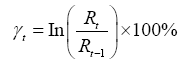where γt denotes the daily logarithmic return at day t,Rt represents the daily return at day t and In(.) represents the natural logarithmic function.

The volatility of the financial returns over the period was examined. From the histogram of returns and tests for normality, we assess the presumption that financial returns have fat tails and deviate from the normal distribution. We initially test the stationarity of the data using the ADF test.

In order to apply the extreme value method to any data, Coles et al.  emphasizes that it is a strong requirement for the data to be independent and identically distributed (iid). To check for this iid requirement, we employed the Box-Ljung test for autocorrelation. In addition to this, a Lagrangian Multiplier (LM) test was performed to test the data for the presence of autoregressive conditional heteroscedastic (ARCH) effects.

Statistical Analysis Software (SAS) 9.3 and R Statistical Software 3.4.1 were used to analyze the data. AR-GARCH modeling was performed in SAS using PROC ARIMA and PROC AUTOREG, while the application of extreme value theory and fitting of GPD model to the exceedances were completed using the extRemes, evir, and qrm packages in R.

ARMA-GARCH model fitting

To produce a complete iid process with relatively no autocorrelation terms and no heteroscedastic effects, different combinations of Autoregressive Moving Average-Generalized Autoregressive Conditional Heteroscedasticity (ARMA-GARCH) models were fitted McNeil and Frey . The best fitting model was identified based on the Akaike information criterion (AIC) and Bayesian information criterion (BIC) values.

Based on the ADF test, it was found that the returns series for BDO, MEG, SCC, SM, and URC are fairly stationary along the mean. An ARMA model is fitted to the data then the Lagrangian Multiplier (LM) test was performed. If the LM test reveals the presence of significant ARCH effects, an ARMA-GARCH model was fitted to the data.

The residuals from the chosen ARMA-GARCH model were extracted with their corresponding conditional variances. A standardized iid series was calculated as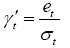where et is the residual term at time t and σt is the corresponding conditional standard deviation at time t.

Extreme value modeling

The first step in the application of POT approach of the EVT is the selection of appropriate threshold levels for the tails of the distribution. So far, there is no algorithm with a satisfactory performance for the selection of the threshold u available Magnou . It is for this reason that graphical approaches are used to select the threshold u by using hill plots and shape parameter plots. The use of the semi-automatic threshold selection sel. threshold function in R was introduced in this study.

After setting the threshold, a GDP model was fitted to each of the tails of the standardized series. The adopted EVT model is that of the GPD. Not only does this approach allow reliable estimation of VaR, CVaR, and return level, but it also provides insight on the tail thickness through the fitted value of one of the GPD parameters known as the shape parameter Loh and Stoyanov . Plots of the estimated GPD models fitted as curves against empirical excesses over the selected thresholds and empirical quantiles of excesses against the quintiles of the fitted GPD models in quantile-quantile (Q-Q) plots are examined to evaluate the fit of the model to the series. MLEs of the parameters are calculated.

Calculation of risk measures under EVT

The risk measures VaR and CVaR associated with each of the tails were computed under various αlevels set at α=0.05,0.025,0.01,0.005 using the fitted GDP model. M-year return level estimates were computed for various periods: 1 year, 5 years, 10 years, 20 years, and 50 years. The risk measures were computed using equations. The VaR under normal distribution assumption was also computed for the various levels. The computed VaR under these two methods were compared with the empirical result.

Assessment of VaR models using backtesting

The back testing procedure developed by the Basel Committee on banking supervision was used to assess the accuracy of VaR models using 95% and 99% coverage levels. Data spanning July 1, 2016 to June 30, 2017 were used for back testing in which there are 265 trading days. Since the Basel Committee only released the three-zone approach tresholds for 99% coverage level for a sample size of 250, thresholds for 95% and 99% coverage level and n=265 were computed and presented in Tables A1 and A2.

#### Results and Discussion

This paper applied the EVT approach in analyzing extreme daily returns of BDO, MEG, SCC, SM, and URC. An in-depth analysis of the extreme value methodology applied to the high frequency (daily) returns of the five selected stocks of the PSE is presented.

ARMA-GARCH modeling

The daily logarithmic returns plot for each of the five stocks is presented in Figure 2. It can be observed that the returns series are fairly stationary along the mean. Heteroscedasticity, or the circumstance in which the variability of a variable is unequal over time, is prominent in the daily returns series as expected in a financial time series data. This suggests that an ARMAGARCH model is to be fitted to the data to model the mean and the variance.

Figure 3 illustrates the histogram of the daily returns data. A classical bell-shaped, symmetric histogram for each of the stocks can be seen with most of the frequency counts gathered in the middle and with the counts dying off in the tails. Examination of the histogram of the daily returns illustrates that the stocks have fat tails.

Computed summary statistics from the data indicate that all the returns series have positive means, non-zero coefficient of skewness, and high positive coefficient of kurtosis. Furthermore, Kolmogorov- Smirnov, Cramer von Mises, and Anderson-Darling tests for normality confirm the non-normality of the series (p<0.05). These summary statistics and tests for normality results are shown in detail in Tables A3 and Table A4.

ADF test revealed that the returns series are relatively stationary (p<0.05). The means were modeled using a purely-AR model so that the AUTOREG procedure in SAS may be used to model the variance using GARCH models in the case that conditional heteroscedastic terms are present in the series. Fitted ARMA-GARCH models for each of the selected stocks are presented in Table 2. Corresponding parameter estimates and statistics for the fitted models are tabulated in Table A5. The daily returns of SCC is found to be stationary and can be considered as a complete iid process with relatively no autocorrelation (p<0.05), as indicated by the Box-Ljung test. Significant ARCH effects are present in all the five stocks. Hence, GARCH or ARCH models were fitted.

Stock Subsector Mean Variance
BDO banking and financials AR(2) GARCH(1,1)
MEG property AR(3) ARCH(1)
SCC mining constant mean GARCH(1,1)
SM conglomerate AR(2) ARCH(1)
URC consumer AR(3) GARCH(1,1)

Table 2: Results of the ARMA-GARCH model fitting.

The standardized series for each of the stocks were produced. Autocorrelation and conditional heteroscedasticity tests performed on the standardized series and squared standardized series showed that no autocorrelation existed and no conditional heteroscedastic terms are evident in the series. Hence, the standardized series were considered suitable for the application of EVT. The extRemes, evir, and qrm packages in R were used for the application of EVT.

Extreme value modeling

The first step in the application of EVT is the selection of a threshold. Since both tails will be modeled in this study, a threshold is selected for each of the tails. The interest is to find an appropriate threshold for Generalized Pareto models by fitting the models to a sequence of thresholds in order to find the lowest possible threshold that yields roughly the same parameter estimates as any higher threshold. Previous papers on the application of EVT used the graphical approach for threshold selection. A similar approach is employed in this study through the use of hill plots and GPD shape parameter plots. The threshold selection function in qrm package was introduced in this study, in addition to the traditional graphical approach. Figures 4 and 5 show the hill plots of the 250 order statistics for the right tails and 350 order statistics for the left tail. The threshold is expected to fall within a range where the hill plots are relatively stable. These ranges are indicated by the two vertical lines in each plot.

Upon examination of the GPD shape parameter plots in Figures 6 and 7, it was observed that for both tails the shape plots are relatively steady in the ranges determined from the hill plots. The shape parameters are considered to be stable within the ranges indicated by the vertical lines, which are similar to or narrower than ranges in the hill plots. The ranges for which the hill plots and GPD shape parameter plots are both stable are at (0.6881, 0.7257) for the left tail and (0.8437, 0.8969) for the right tail distributions of BDO, (0.7767, 0.8125) and (1.0939, 1.1208) for MEG, (0.6983, 0.7380) and (0.8116, 0.8583) for SC, (0.5193, 0.7584) and (0.8417, 0.8906) for SM, and (0.5212, 0.5413) and (0.8308, 0.8531) for URC. These ranges were used in the sel.threshold function in R to identify the corresponding threshold within each range.

The calculated threshold values are tabulated in Table 3. It can be observed that higher threshold values were computed for the right tails which suggests that the distribution of losses is more stable compared to the distribution of gains which are more extreme.

Stock Left-tail Right-tail
BDO 0.715 0.895
MEG 0.812 1.11
SCC 0.699 0.829
SM 0.701 0.889
URC 0.521 0.651

Table 3: Computed threshold for the tail distribution of the stocks.

GPD models were fitted to the exceedances with respect to the threshold levels and parameter estimates for the fitted models were calculated. Plots of the estimated GPD models fitted as curves against empirical excesses over the selected thresholds are presented in Figures 8 and 9. The plots show that the estimated GPD models provide a good fit to the extreme values since all the points on the plots lie approximately on the curves with no significant deviations to the curves. Tabulated GPD model parameter estimates and statistics are shown in Table A6.

It can be seen from Figures 8 and 9 that the GPD models provide a good fit to the exceedances for the tails of the returns series for each of the selected stocks. This is also confirmed by the results obtained by fitting the empirical quantiles of excesses against the quantiles of the fitted GPD models in quantile-quantile (QQ) plots shown in Figures 10 and 11. For both tails, the plots do not have significant departures from the straight line. The points corresponding to the largest observations can be observed on the plot but are not considered significant deviations since they are not very distant from the line.

Plots of the fitted GPD models and QQ-plots confirm the adequacy of the fitted models to the tails of the distribution of returns. Risk measures were computed using the fitted GPD models.

Risk measures

The computed VaR and CVaR for each of the tails after fitting the extreme value distribution indicate that at a 95% confidence level, the expected market loss would not be more than 5.95% for BDO, 4.81% for MEG, 4.13% for SCC, 3.76% for SM, and 5.66% for URC for a oneday duration. In the case that the daily loss exceeds these levels, the average loss is expected to be 8.16% for BDO, 5.54% for MEG, 5.11% for SCC, 5.14% for SM, and 7.10% for URC. Similarly, the expected market return for one-day duration is expected to be at most 4.78% for BDO, 6.52% for MEG, 5.21% for SCC, 4.46% for SM, and 4.27% for URC. If the daily gain exceeds these levels, an average gain is expected to be 6.33% for BDO, 9.51% for MEG, 6.22% for SCC, 6.13% for SM, and 5.44% for URC. Similar interpretation may be done for the computed risk measures for higher quantiles. The computed VaR and CVaR values under EVT framework for other levels of α are summarized in Table A7.

Alternatively, the above results also imply that for an investment of Php 1 million in these stocks, the expected loss over one trading day will not exceed Php 59,500 for BDO, Php 48,100 for MEG, Php 41,300 for SCC, Php 37,600 for SM, and Php 56,600 for URC, with a 95% confidence level. For the same investment and with the same confidence level, the expected gain over one trading day will not exceed Php 47,800 for BDO, Php 65,200 for MEG, Php 52,100 for SCC, Php 44,600 for SM, and Php 42,700 for URC.

A comparison of the risk measures between the right tails and left tails indicate that from July 1, 2006 to June 30, 2016, investments in BDO and URC have been exposed to more extreme losses than extreme gains, as evidenced by the greater VaR values for the left tails compared to the right tails. The opposite may be said about investments in MEG, SCC, and SM, which have been exposed to more extreme gains rather than extreme losses.

One of the risk measures, return level, determine the maximum gain or loss of each of the stocks. For illustrative purposes, the return levels for BDO are interpreted as follows: a maximum loss of 8.54% is expected once every year, a maximum loss of 12.15% is expected every five years, 14.13% every 10 years, 16.43% every 20 years, and 20.03% every 50 years; a maximum gain of 8.27% is expected once every year, a maximum gain of 11.06% is expected every five years, 12.56% every 10 years, 14.19% every 20 years, and 16.72% every 50 years. Similar means of interpretation may be done for the return level estimates for the other stocks. Return levels for other stocks were computed and tabulated in Table A8.

A comparison of the calculated VaR under EVT and normal distribution assumption against the empirical results revealed that for lower quantiles or higher levels, the two methods tend to slightly overestimate the VaR. However, it was observed that for higher quantiles or lower α levels, VaR estimates under normal distribution assumption significantly underestimated the VaR. On the other hand, VaR estimates under EVT converges to the empirical result. VaR values under normal distribution assumption and empirical results are shown in Table A8.

Backtesting

To assess the accuracy of the VaR models, backtesting using the three-zone approach developed by the Basel Committee was performed. Results of the backtesting procedure are found in Table 4 for the 99% and 95% coverage levels. Zone classifications for the number of exceedances were based on the calculated values for the three-zone approach in Tables A1 and A2. Backtesting results show that all the VaR models under EVT are classified under the Green zone which indicates high probability of model accuracy.

A similar framework for backtesting was applied to VaR under normal distribution assumption. Results are shown in Table 4. Inspection of Table 5 shows that there are more exceptions for VaR under normal distribution assumption compared to EVT.

Stock Number of exceptions Zone  Zone
99% 95% 99% 95%
BDO Left 0 0 Green Green
Right 0 0 Green Green
MEG Left 2 7 Green Green
Right 0 0 Green Green
SCC Left 2 4 Green Green
Right 0 4 Green Green
SM Left 2 4 Green Green
Right 1 7 Green Green
URC Left 0 1 Green Green
Right 1 6 Green Green

Table 4: Backtesting results for VaR under EVT.

Stock Number of exceptions Zone
99% 95% 99% 95%
BDO Left 6 9 Yellow Green
Right 1 0 Green Green
MEG Left 1 11 Yellow Green
Right 11 19 Green Yellow
SCC Left 2 8 Yellow Green
Right 6 9 Green Green
SM Left 5 12 Yellow Green
Right 10 15 Green Green
URC Left 1 3 Yellow Green
Right 2 7 Green Green

Table 5: Backtesting results for VaR under normal distribution assumption.

It can also be seen that not all VaR models under the normal distribution assumption were classified under the Green zone, specifically for the 99% coverage level, as observed in left tail model for BDO and right tail models for MEG, SCC, and SM. This implies that the VaR approach using the assumption of normality was not able to capture extreme losses, and therefore, underestimated tail risk.

#### Conclusion

The main objective of this paper is to examine the application of EVT in measuring financial risk of the selected Philippines stocks BDO, MEG, SCC, SM, and URC. Results of the study showed that the daily returns series of these five stocks deviate from the normal distribution and are asymmetric in nature. Hence, the EVT model is found to be more appropriate to fit to the tails of the distribution of returns.

Preference for the conditional EVT approach was due to the observed volatility in the data and previous studies [25,31] which concluded that conditional EVT is better than the unconditional approach in financial risk applications. The paper employed the POT approach of the EVT, which fitted a GPD model to excesses above a threshold u for each of the tails of the distributions of the selected stocks. As concluded in studies by Genkay and Selkuk (2004) and Krehbiel and Adkins  that the left and right tails behave differently and should be treated separately, a similar realization was observed in this study. The GPD models were found to provide a good fit to the data as evidenced by the plots of empirical exceedances with the fitted GPD model and the QQ plots.

Risk measures under the EVT framework revealed that stock investments in BDO and URC have been exposed to more extreme losses than extreme gains, while MEG, SCC, and SM have been exposed to more extreme daily returns. VaR and CVaR for various levels were computed, as well as M-year return levels for various periods. Assessment of VaR models using the three-zone approach developed by the Basel Committee on Banking Supervision yielded positive results. The VaR models under the EVT framework for each of the tails of the selected stocks fall under the green zone of backtesting, indicating high probability of model accuracy. A comparison of the calculated VaR under EVT and normal distribution assumption against the empirical results showed that as becomes smaller, VaR estimates under normal distribution assumption tend to underestimate VaR while estimates under EVT approach the empirical VaR values.

This study was able to illustrate the use of the POT approach of EVT in modeling tail returns of selected Philippine stocks. The possibility of utilizing the semi-automatic threshold selection function in R was also explored. Risk measures VaR, CVaR, and return level were computed and VaR estimates under EVT and under the normal distribution assumption were compared with empirical results. The accuracy of the computed VaR estimates were also assessed through backtesting using Basel committee three-zone approach. With the results of this study, it can be concluded that the POT approach of conditional EVT can be utilized in measuring financial risk associated with five selected Philippine stocks: BDO, MEG, SM, SCC, and URC.

#### Recommendations

In a study by Suaiso and Mapa , the VaR model under EVT was compared with the Risk Metrics model developed by J.P. Morgan and different ARMA-GARCH type models. The RiskMetrics model employs various time series techniques in modeling the mean and variance of returns. However, ARMA-GARCH type models do not forecast VaR. The models forecast the returns and conditional variance of the time series. The returns are forecasted using ARMA models, while the volatility is forecasted using models such as GARCH, exponential GARCH (EGARCH), GARCH-in-mean (GARCH-M), and integrated in variance GARCH (IGARCH). These volatility models are said to capture volatility clustering in financial time series data.

Hence, in addition to VaR models under the normal distribution assumption, VaR models under the RiskMetrics approach and ARMAGARCH approach may also be included for comparison with EVTbased VaR models.

In assessing VaR models, banks look into a model’s conservatism, accuracy, and efficiency. A VaR model is considered conservative if it systematically produces high estimates of risk relative to other models. Accuracy can be assessed by analyzing the number of times the VaR estimates are lower than the losses and the magnitude of those losses. An efficient VaR model provides sufficient conservatism .

One measure of model conservatism is the mean relative bias (MRB) statistic Hendricks . It tests whether different VaR models produce risk estimates of similar average size. For model accuracy, backtesting using the Basel Committee three-zone approach is the standard practice. Likelihood ratio (LR) tests, in contrast, can be used to assess if the model is inaccurate . Therefore, the use of MRB statistic and LR tests is recommended in assessing model adequacy for future research.

In this study, the EVT was applied to five selected stocks listed in the Philippine stock exchange. Applying these methods to a more representative sample of Philippine stocks may also be considered in subsequent studies.

#### References

Citation: Velasco AAF, Lapuz DKP (2018) Extreme Value Modelling for Measuring Financial Risk with Application to Selected Philippine Stocks. J Appl Computat Math 7: 404. DOI: 10.4172/2168-9679.1000404

Copyright: © 2018 Velasco AAF, et al. This is an open-access article distributed under the terms of the Creative Commons Attribution License, which permits unrestricted use, distribution, and reproduction in any medium, provided the original author and source are credited.

Select your language of interest to view the total content in your interested language

##### Recommended Journals
Viewmore
###### Article Usage
• Total views: 2310
• [From(publication date): 0-2018 - Nov 21, 2019]
• Breakdown by view type
• HTML page views: 2199Can't read the image? click here to refresh